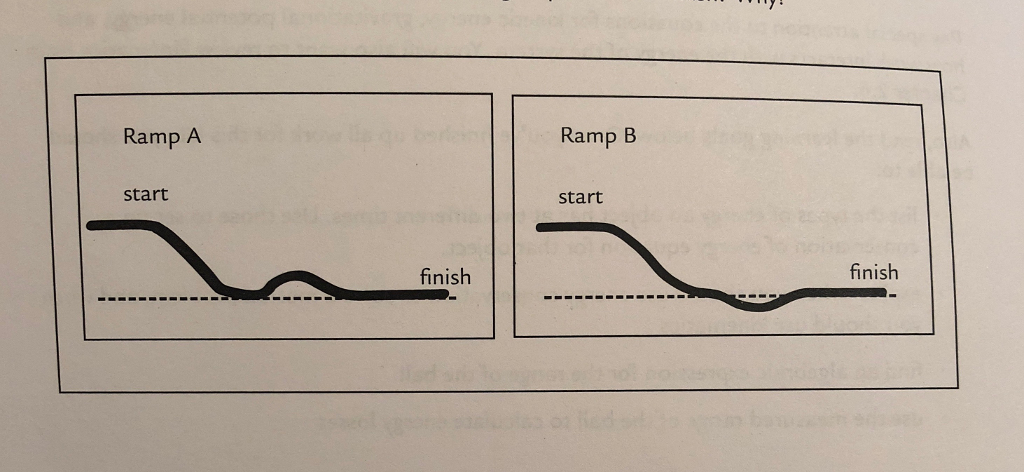# Problem: You release a frictionless cart at the top of each of the 2 ramps. BOTH CARTS ARE RELEASED FROM REST. The ramps have the same height as each other at the starting and finishing points. One has a small bump up then down before the end. The other has a small bump down then up. Which cart has the larger speed at the finish and why?

###### FREE Expert Solution

Energy conservation,

$\overline{){\mathbf{m}}{\mathbf{g}}{{\mathbf{h}}}_{{\mathbf{0}}}{\mathbf{+}}\frac{\mathbf{1}}{\mathbf{2}}{\mathbf{m}}{{{\mathbf{v}}}_{{\mathbf{0}}}}^{{\mathbf{2}}}{\mathbf{=}}{\mathbf{m}}{\mathbf{g}}{{\mathbf{h}}}_{{\mathbf{f}}}{\mathbf{+}}\frac{\mathbf{1}}{\mathbf{2}}{\mathbf{m}}{{\mathbf{v}}}_{{\mathbf{2}}}}$

The initial and final heights for the two carts are the same.

83% (449 ratings)###### Problem Details

You release a frictionless cart at the top of each of the 2 ramps. BOTH CARTS ARE RELEASED FROM REST. The ramps have the same height as each other at the starting and finishing points. One has a small bump up then down before the end. The other has a small bump down then up. Which cart has the larger speed at the finish and why?Frequently Asked Questions

What scientific concept do you need to know in order to solve this problem?

Our tutors have indicated that to solve this problem you will need to apply the Conservative Forces & Inclined Planes concept. You can view video lessons to learn Conservative Forces & Inclined Planes. Or if you need more Conservative Forces & Inclined Planes practice, you can also practice Conservative Forces & Inclined Planes practice problems.

What professor is this problem relevant for?

Based on our data, we think this problem is relevant for Professor Leduc's class at SDSU.